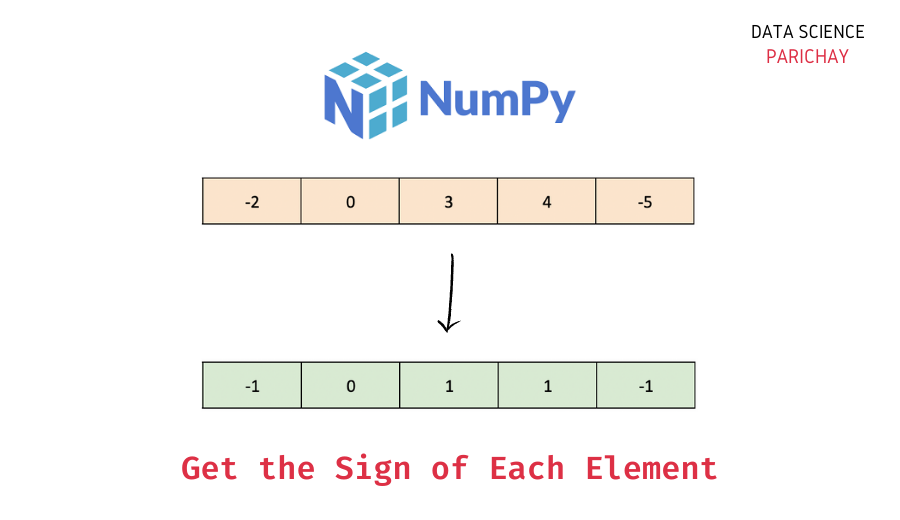# Numpy – Get the Sign of Each Element in Array

The Numpy library in Python comes with a number of built-in functions to perform common mathematical operations on arrays. In this tutorial, we will look at one such function that helps us get the elementwise sign (positive, negative, or zero) of a Numpy array with the help of some examples.

## How to get the sign of values in Numpy array?

You can use the `numpy.sign()` function to get the sign of each element in a Numpy array. Pass the array as an argument.

The following is the syntax –

`numpy.sign(ar)`

It returns an array containing the sign of each element. A positive element has sign 1, a negative element has sign -1, and zero has sign 0. (We’re basically applying the mathematical signum function on each element).

Let’s now look at a step-by-step example of using the `numpy.sign()` function.

### Step 1 – Create a Numpy array

First, we will create a Numpy array that we will use throughout this tutorial.

```import numpy as np

# create numpy array
ar = np.array([1, -3, 4, 0, 5, -2, -7])
# display the array
print(ar)```

Output:

`[ 1 -3  4  0  5 -2 -7]`

Here, we used the `numpy.array()` function to create a Numpy array containing some numbers. You can see that this array contains both positive and negative numbers (along with a 0).

📚 Data Science Programs By Skill Level

Introductory

Intermediate ⭐⭐⭐

🔎 Find Data Science Programs 👨‍💻 111,889 already enrolled

Disclaimer: Data Science Parichay is reader supported. When you purchase a course through a link on this site, we may earn a small commission at no additional cost to you. Earned commissions help support this website and its team of writers.

### Step 2 – Get the sign of every element using `numpy.sign()`

To get the sign of each element in a Numpy array, pass the array as an argument to the `numpy.sign()` function.

Let’s get the sign of elements in the array created above.

```# get the sign of each element
np.sign(ar)```

Output:

`array([ 1, -1,  1,  0,  1, -1, -1])`

We get a Numpy array with the sign of each element in the array `ar`.

The `numpy.sign()` function works similarly on higher-dimensional arrays. For example, let’s apply this function to a 2D array of some numbers.

```# create 2D numpy array
ar = np.array([[1, -3, 4],
[0, 5, -2],
[-6, 8, -7]])
# get the sign of each element
np.sign(ar)```

Output:

```array([[ 1, -1,  1],
[ 0,  1, -1],
[-1,  1, -1]])```

You can see that we get the sign of each element in the 2D array.

For more on the `numpy.sign()` function, refer to its documentation.

You might also be interested in –

•